StatLect

# Autocorrelation

Autocorrelation is the coefficient of linear correlation between two terms of a sequence of random variables.

Autocorrelation is also called serial correlation.## Definition

The following is formal definition.

Definition Letbe a sequence of random variables. The autocorrelation coefficient between two terms of the sequenceandisIn other words, the autocorrelation coefficient is just the coefficient of linear correlation between two random variables belonging to the same sequence.

Note that the covarianceis called autocovariance.

## Autocorrelation and weakly stationary sequences

Remember that a sequence of random variables is said to be covariance stationary (or weakly stationary) if and only if:

• all the terms of the sequence have the same mean:• the covariance between any two terms of the sequence depends only on on how far apart they are located from each other, and not on where they are located in the sequence:The second of these two properties implies that all the random variables in the sequence have the same variance:because.

When a sequence is covariance stationary, the autocorrelation coefficient between two terms of the sequenceanddepends only on:We denote it by:and we call it autocorrelation at lag(the distancebetween two terms of the sequence is called lag).

## Sample autocorrelation

When we observe the firstrealizations of a sequence, we can compute the sample autocorrelation at lag: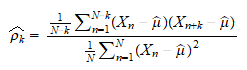whereis the sample meanIfis covariance stationary, then the numerator ofis a consistent estimator ofand the denominator is a consistent estimator of. As a consequence,is a consistent estimator of the autocorrelation at lag.

## Autocorrelation function

The autocorrelation function (ACF) is the function that maps lags to autocorrelations, that is,is considered as a function of(see the examples below).

When the mapping is from lags to sample autocorrelations, then we call it sample ACF.

## ACF plots

An ACF plot is a bar chart (or a line chart) that plots the autocorrelation function:

• lags are on the x-axis;

• the autocorrelations corresponding to the lags are on the y-axis.

## Examples

Let's look at some examples of ACF and ACF plots.

### Example 1 - ACF of an AR(1) autoregressive process

Suppose thatis a covariance stationary sequence such thatwhereis a constant andis an IID sequence of standard normal random variables (zero mean and unit variance).

Such a sequence is called an autoregressive process of order 1, or AR(1) process (the order is the maximum lag of the sequence on the right hand side of the equation).

Note thatwhere we have performed recursive substitutions ofwith.

By using this expression for, we can easily derive the autocovariance at lag:where: in stepsandwe have used the bilinearity of the covariance operator and in stepwe have used the facts that 1) the covariance of a random variable with itself is equal to its variance; 2) the covariance betweenandis zero for anybecausedepends only onforand the sequenceis IID.

Thus, the autocorrelation at lagis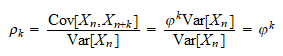The following ACF plots show the autocorrelation function for different values of.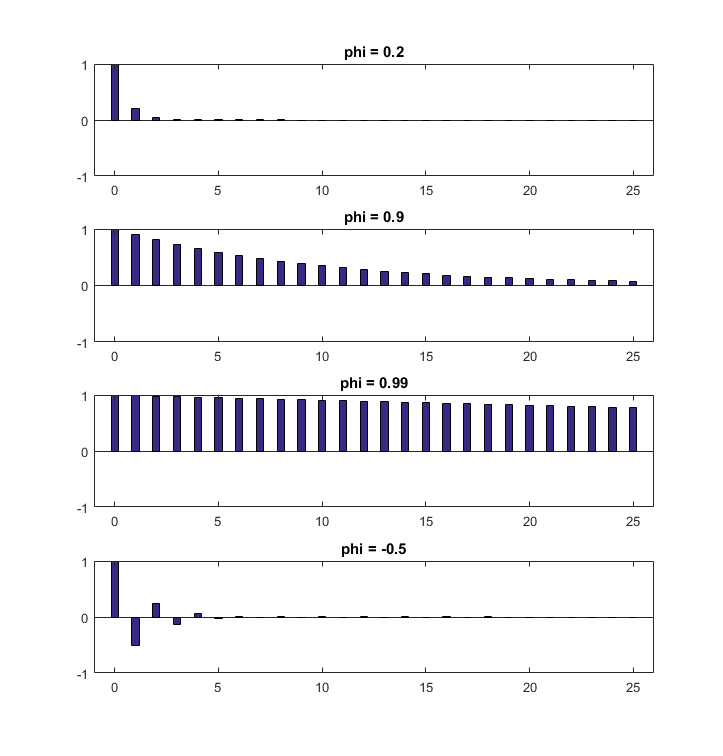### Example 2 - Sample autocorrelation

In this example, we show what a sample ACF looks like.

We generate, via Monte Carlo simulations, 200 realizations for each of the four AR(1) processes whose ACFs have been plotted above. The realizations are plotted below.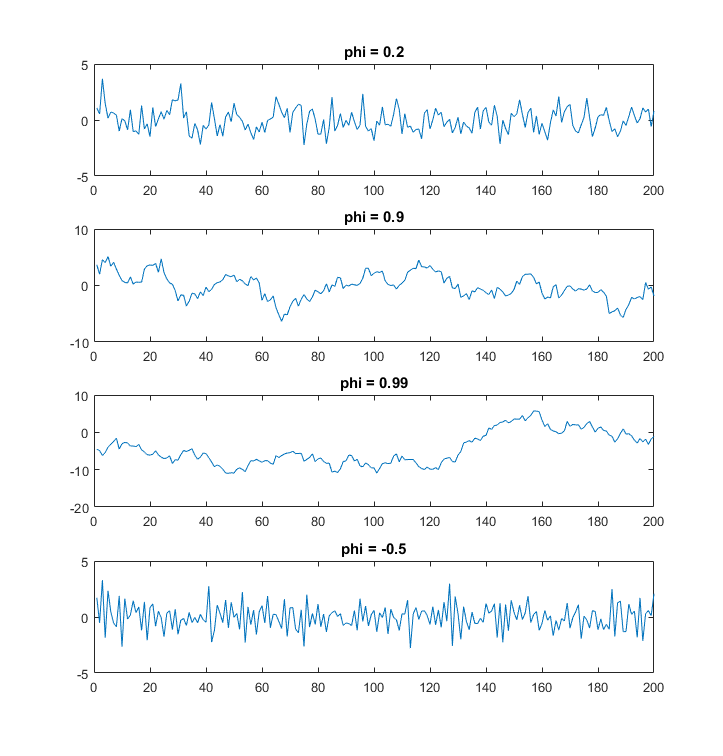We then compute their sample ACFs, which are plotted below.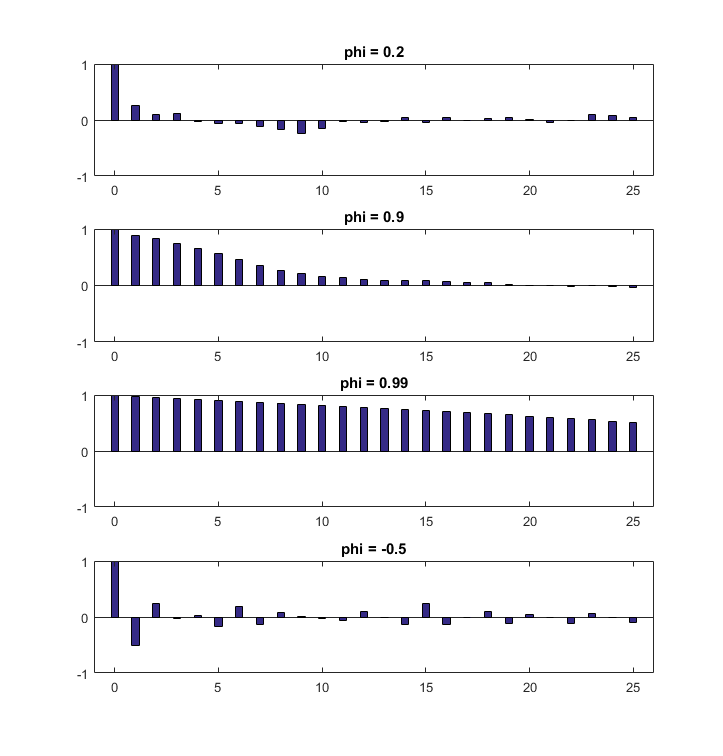These are the sample versions of the ACFs shown in Example 1. As the sample autocorrelations are noisy estimates of the true autocorrelations, these ACFs do not coincide with those shown in Example 1.